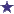# Percentage Strength Volume in Volume Dilution Pharmacy Math Question

by Ray
(McKinleyville, CA, USA)

I am a math idiot and I'm sure this is probably an easy question but here it is. What is the percent strength of a solution that is made by adding 200mL of sterile water to 600mL of a 25% solution?

### Comments for Percentage Strength Volume in Volume Dilution Pharmacy Math Question

Average RatingRatingby: David One quarter (25%) of 600 = 150

 Ratingneed more explanation by: Anonymous please explain going from 25%/600ml to 150/600ml, thanks

 Rating25% of volume is added to the solution; and percentage strength is diluted by 25% by: David First of all, thank you, Ray, for this question! Next, we calculate the amount (in MG) of diluent in the solution: 25%/600ml = 150mg/600mL Currently we have 150mg solute in 600mL solution. Add the 200mL to the solution, which dilutes it... 150mg/800mL = 18.75% is the percentage strength of the newly diluted solution. 25% of 25% = 6.25% 25% - 25% = 18.75%